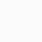## Trigonometry Formulas For Class 10

Trigonometry is an essential part of math. Trigonometry is important in terms of board examinations. Therefore, to solve the problems of trigonometry, it is necessary to remember the formulas. For this reason, in this article on All Trigonometry Formulas For Class 10, 11, 12, we have shown how to remember through logic.

### Trigonometric Ratio

In a right-angled triangle, the opposite side of a given angle is perpendicular, the opposite side of the right angle is the hypotenuse and the third side is the base.

In ΔABC,
∠=90°,∠C=θ

∴ The side AC is the hypotenuse,
AB is perpendicular
and BC is the base.

From the Pythagoras theorem,

## Relationship between arms and angles

Finding the formulas of cosec, sec, and a cot can be obtained by reversing the formulas of sin, cos, and tan.

This means that the formula of cosec will be the inverse of sin, the formula of sec will be the inverse of sec, and the procedure of cot will be the inverse of tan. The numerator is replaced by the numerator and denominator.

#### Ratios Of Particular Angle Trigonometry Formulas For Class 10

The ratio formula of trigonometry is very important from this point of view. Which is important for all classes of students. In The Ratio Trigonometry Formulas For Class 10, you can remember sin, cos, and tan value. and the other three are opposite like this sin is to Reverse cosec. cos is Reverse to sec and tan Reverse to cot

### The trigonometrical function of the sum

There are definite relations between trigonometric functions of two angles of a triangle.

In the article, “Trigonometry Formulas For Class 10” of math is given. It is based on these relations, especially their sum and difference.

### 4 Responses

1.Rohit says:

Sir please check your trignometry squares formulei specially tan square and cot square and kindly correct it thank u.

2.Sandesh says:

isn’t it cosec2a-cot2a=1
where,2=square

3.Buy Best Proxies says:

some really interesting points you have written.

4.Redmi Note ⁹ Pro Max says:

Thank you so much sir